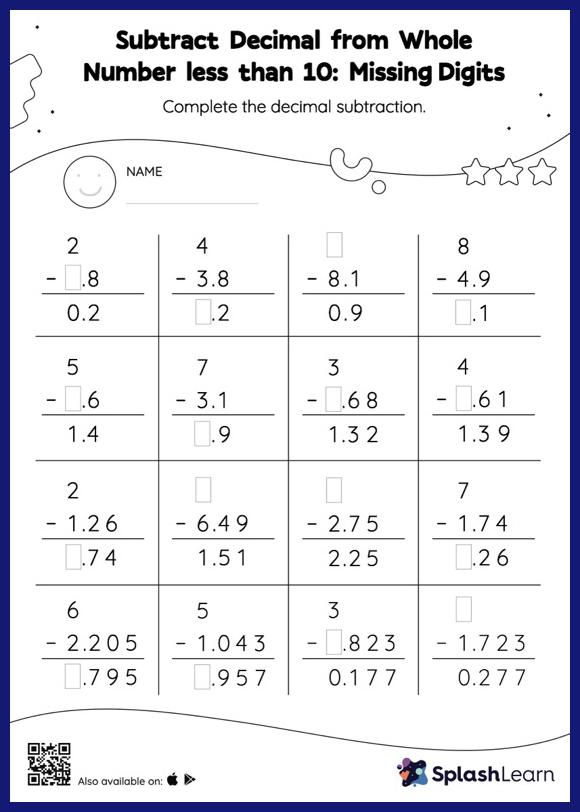# Subtract Decimal from Whole Number less than 10: Missing Digits Worksheet

Home > Subtract Decimal from Whole Number less than 10: Missing DigitsGive your little one some practice building subtraction skills with this subtract decimal from whole number less than 10 worksheet. When subtracting decimals on this worksheet, students align the decimal points and use zero as a placeholder. Then to find the missing number in the subtract decimal from whole number less than 10 worksheet, students then apply the relationship between addition and subtraction. In each problem, the numbers are laid out in the vertical format. In this format, pair of digits in each successive place are tackled one by one. This helps students follow a structured approach.